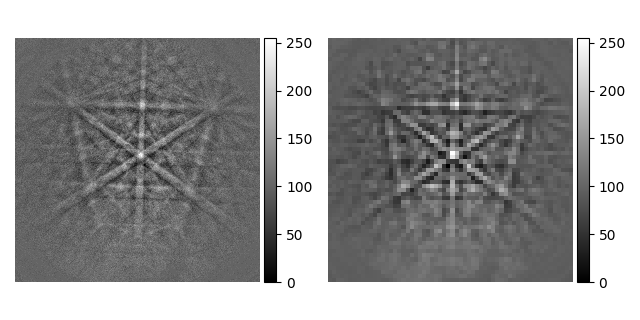# Pattern binning#

This example shows how bin `EBSD` patterns using HyperSpy’s `rebin()`.

```import hyperspy.api as hs
import kikuchipy as kp
import numpy as np

print(s)

s.remove_static_background(show_progressbar=False)
```

Out:

```<EBSD, title: si_in Scan 1, dimensions: (|480, 480)>
```

Rebin by passing the new shape (use `(1, 1, 60, 60)` if binning a 2D map)

```s2 = s.rebin(new_shape=(60, 60))

_ = hs.plot.plot_images(
[s, s2],
axes_decor="off",
tight_layout=True,
label=None,
colorbar=False,
)
```Rebin by passing the new:old pixel ratio (again, use `(1, 1, 8, 8)` for a 2D map)

```s3 = s.rebin(scale=(8, 8))
print(np.allclose(s2.data, s3.data))
```

Out:

```True
```

Notice how `rebin()` casts the data to `uint64`, increasing the memory use by a factor of eight (so be careful…)

```print(s.data.dtype)
print(s3.data.dtype)
```

Out:

```uint8
uint64
```

Rescale intensities to the initial data type

```s3.rescale_intensity(dtype_out=s.data.dtype, show_progressbar=False)
print(s3.data.dtype)
```

Out:

```uint8
```

Total running time of the script: ( 0 minutes 3.173 seconds)

Estimated memory usage: 19 MB

Gallery generated by Sphinx-Gallery